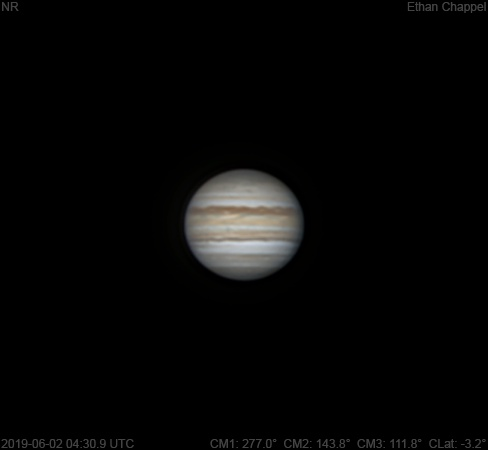# Jupiter 2019-06-02 04:30 UTC

CM1: 277.00°

CM2: 143.80°

CM3: 111.80°

CLat: -3.20°

Description

I wasn't expecting to image tonight, but I went outdoors to see the sky clearing to the south. The stars were twinkling and the sky could've clouded over at any time, so we skipped the C14 and I pulled out the 90mm Mak for a quick setup. Sky conditions were very good! I believe this is one of my best photos from this scope!

Notes:

• The North Temperate Belt is thin. The southern edge is orange while the north is dark.
• The North Equatorial Belt appears to have a few new rifts.
• The Equatorial Zone, except for the southern edge, is still orange.
• The South Equatorial Belt appears to have a dark spot on its northern edge approaching the central meridian.
• The SEBs is dark compared to the rest of the belt.
• The South Polar Red Spot is past the CM.

Equipment

ZWO ASI224MC

Orion Apex 90mm

Logs
```FireCapture v2.6  Settings
------------------------------------
Observer=Ethan Chappel
Camera=ZWO ASI224MC
Filter=RGB
Profile=Jupiter
Diameter=45.80"
Magnitude=-2.60
CMI=277.3° CMII=144.1° CMIII=112.2°  (during mid of capture)
FocalLength=1200mm (F/4)
Resolution=0.65"
Filename=2019-06-02-0431_6-EC-RGB-Jup.ser
Date=2019_06_02
Start=04_30_25.584
Mid=04_31_40.584
End=04_32_55.585
Start(UT)=04_30_25.584
Mid(UT)=04_31_40.584
End(UT)=04_32_55.585
Duration=150.001s
Date_format=yyyy_MM_dd
Time_format=HH_mm_ss
LT=UT -6h
Frames captured=9593
File type=SER
Binning=no
Bit depth=8bit
Debayer=yes
DebayerAlgorithm=NearestNeighbor
BayerPattern=RG
ROI=464x460
ROI(Offset)=0x0
FPS (avg.)=63
Shutter=15.00ms
Gain=250 (41%)
HardwareBin=off
Gamma=50 (off)
FPS=100 (off)
AutoExposure=off
AutoHisto=75 (off)
HighSpeed=off
SoftwareGain=10 (off)
WBlue=95 (off)
AutoGain=off
USBTraffic=100 (off)
Brightness=1 (off)
WRed=52 (off)
Histogramm(min)=0
Histogramm(max)=0
Histogramm=0%
Noise(avg.deviation)=n/a
AutoAlign=false
PreFilter=none
Limit=150 Seconds
Sensor temperature=33.3°C
FireCapture v2.6  Settings
------------------------------------
Observer=Ethan Chappel
Camera=ZWO ASI224MC
Filter=RGB
Profile=Jupiter
Diameter=45.80"
Magnitude=-2.60
CMI=274.2° CMII=141.0° CMIII=109.0°  (during mid of capture)
FocalLength=1150mm (F/4)
Resolution=0.67"
Filename=2019-06-02-0426_5-EC-RGB-Jup.ser
Date=2019_06_02
Start=04_25_15.154
Mid=04_26_30.159
End=04_27_45.165
Start(UT)=04_25_15.154
Mid(UT)=04_26_30.159
End(UT)=04_27_45.165
Duration=150.011s
Date_format=yyyy_MM_dd
Time_format=HH_mm_ss
LT=UT -6h
Frames captured=9594
File type=SER
Binning=no
Bit depth=8bit
Debayer=yes
DebayerAlgorithm=NearestNeighbor
BayerPattern=RG
ROI=464x460
ROI(Offset)=0x0
FPS (avg.)=63
Shutter=15.00ms
Gain=250 (41%)
HardwareBin=off
Gamma=50 (off)
FPS=100 (off)
AutoExposure=off
AutoHisto=75 (off)
HighSpeed=off
SoftwareGain=10 (off)
WBlue=95 (off)
AutoGain=off
USBTraffic=100 (off)
Brightness=1 (off)
WRed=52 (off)
Histogramm(min)=0
Histogramm(max)=0
Histogramm=0%
Noise(avg.deviation)=n/a
AutoAlign=false
PreFilter=none
Limit=150 Seconds
Sensor temperature=33.3°C
FireCapture v2.6  Settings
------------------------------------
Observer=Ethan Chappel
Camera=ZWO ASI224MC
Filter=RGB
Profile=Jupiter
Diameter=45.80"
Magnitude=-2.60
CMI=279.1° CMII=145.9° CMIII=113.9°  (during mid of capture)
FocalLength=1150mm (F/4)
Resolution=0.68"
Filename=2019-06-02-0434_6-EC-RGB-Jup.ser
Date=2019_06_02
Start=04_33_23.934
Mid=04_34_38.937
End=04_35_53.940
Start(UT)=04_33_23.934
Mid(UT)=04_34_38.937
End(UT)=04_35_53.940
Duration=150.006s
Date_format=yyyy_MM_dd
Time_format=HH_mm_ss
LT=UT -6h
Frames captured=9594
File type=SER
Binning=no
Bit depth=8bit
Debayer=yes
DebayerAlgorithm=NearestNeighbor
BayerPattern=RG
ROI=464x460
ROI(Offset)=0x0
FPS (avg.)=63
Shutter=15.00ms
Gain=250 (41%)
HardwareBin=off
Gamma=50 (off)
FPS=100 (off)
AutoExposure=off
AutoHisto=75 (off)
HighSpeed=off
SoftwareGain=10 (off)
WBlue=95 (off)
AutoGain=off
USBTraffic=100 (off)
Brightness=1 (off)
WRed=52 (off)
Histogramm(min)=0
Histogramm(max)=0
Histogramm=0%
Noise(avg.deviation)=n/a
AutoAlign=false
PreFilter=none
Limit=150 Seconds
Sensor temperature=33.3°C
```